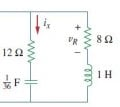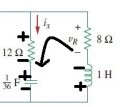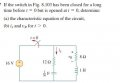# KVL for a RLC circuit given current direction and polarity of a resistor?

#### AristotleX

Joined Nov 1, 2015
2
Here is the diagram of the circuit:I am actually looking for the voltage across the inductor (VL), so I decided the best approach in finding it is through Kirchoff's Voltage Law.
Being given the polarity of the resistor, should I follow the passive sign convention for the other components in the circuit while doing KVL...if I decide to do a loop in the counterclockwise direction?

I understand that current conventionally flows through the positive terminal to the negative.
So would it be correct if I label the circuit like this, if I did a KVL mesh counterclockwise?:Any help would be appreciated, thank you.

#### Jony130

Joined Feb 17, 2009
5,439
But what is the purpose of doing KVL for a component that are connected in parallel in the circuit ? Do you know that components in parallel are connected between same two points. Hence, voltage is the same across parallel components. Therefore :
VR_8Ω + VL = VR_12 Ω + VC

#### AristotleX

Joined Nov 1, 2015
2
But what is the purpose of doing KVL for a component that are connected in parallel in the circuit ? Do you know that components in parallel are connected between same two points. Hence, voltage is the same across parallel components. Therefore :
VR_8Ω + VL = VR_12 Ω + VC
Oh sorry I wasn't clear. This is actually the problem itself: But in doing part B, I needed to find the voltage across the inductor to look for my general equation for ix(t). So in consideration for when the switch is open, I only took account of the series RLC circuit.
My concern was whether the polarities that I've shown above were correct? Thank you for your reply Jony.#### WBahn

Joined Mar 31, 2012
28,174
You can choose things however you like to solve the problem. You can completely ignore the ix and vr completely and just find the current in the loop as a function of time after the switch opens. Then all you need to do is use that current to find ix and vr, taking the polarities shown into account.